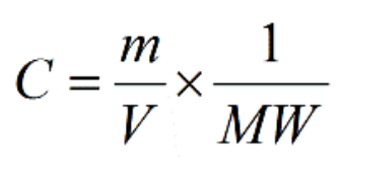# What is the molar oconcentration of 30g KCl and 30g of CaCl2 added to 50 dL of DI water?a.) what is the molar concerntration of Ca2+b.) what is the molar concentration of K+c.) what is the molar concentration of Cl-d.) what is the osmolarity of this solution?e.) what is the tonicity of this solution?

Question
99 views

What is the molar oconcentration of 30g KCl and 30g of CaCl2 added to 50 dL of DI water?

a.) what is the molar concerntration of Ca2+

b.) what is the molar concentration of K+

c.) what is the molar concentration of Cl-

d.) what is the osmolarity of this solution?

e.) what is the tonicity of this solution?

check_circle

Step 1

Formula to find the molar concentration isHere, c is the molar concentration

m is the mass of the solute in grams

v is the volume of the solution in liters

MW is the molecular weight in g/mol.

50 dl of distilled water is equal to 5L.

Step 2

To find the molar concentration of Ca2+

Molecular weight of Ca2+ is 40.08 g/mol

C = (30/5) × (1/ 40.08)

= 6 × 0.02495

= 0.1497

Molar concentration of Ca2+ is 0.1497 mol/L

Step 3

To find the molar concentration of K+

Molecular weight of K+ is 39.0983 g/mol

C = (30/5) × (1/ 39.0983)

&nbs...

### Want to see the full answer?

See Solution

#### Want to see this answer and more?

Solutions are written by subject experts who are available 24/7. Questions are typically answered within 1 hour.*

See Solution
*Response times may vary by subject and question.
Tagged in

### Biology